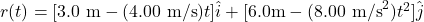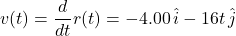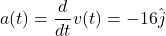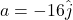## . An object has a position given by ~r(t) = [3.0 m − (4.00 m/s)t]ˆı + [6.0 m − (8.00 m/s2 )t 2 ]ˆ , where all quantities are in SI units. W

Question

. An object has a position given by ~r(t) = [3.0 m − (4.00 m/s)t]ˆı + [6.0 m − (8.00 m/s2 )t 2 ]ˆ , where all quantities are in SI units. What is the magnitude of the acceleration of the object at time t = 5.00 s? (a) 8.94 m/s 2 (b) 0.00 m/s 2 (c) 16.0 m/s 2 (d) 8.00 m/s 2 (e) 4.00 m/s 2

in progress 0
6 months 2021-08-28T11:07:40+00:00 1 Answers 2 views 0

(c) 16 m/s²

Explanation:

The position is.

The velocity is the first time-derivative of r(t).The acceleration is the first time-derivative of the velocity.Since a(t) does not have the variable t, it is constant. Hence, at any time,Its magnitude is 16 m/s².